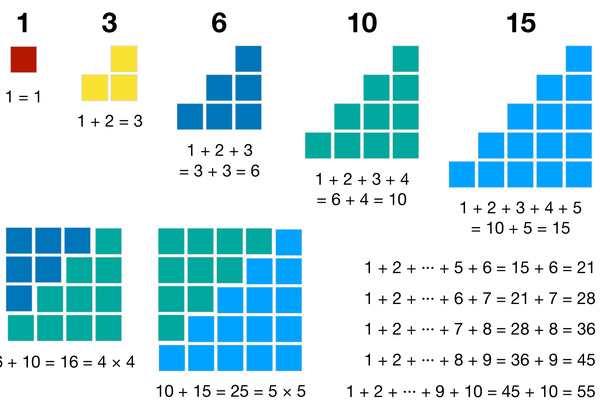# Number Blocks: Triangular NumbersTriangular numbers, or triangle numbers, are a sequence of numbers that can be represented by a pattern of objects arranged in an equilateral triangle. The numbers of this sequence are 1, 3, 6, 10, 15, 21, 28, 36, and so on. The n-th triangular number is equal to the sum of the n natural numbers from 1 to n. The concept of triangle numbers is introduced to kids in secondary school. But this knowledge is quite useful for kids in fifth grade and above to solve many other math problems. Learning triangle numbers can help kids develop their ability to recognize patterns, understand number formulas, and discover relationships between geometric shapes and numbers.

This worksheet shows the first few triangle numbers illustrated by number blocks and their corresponding calculations. It is easy to see the pattern that one more block is added to the next row, and the sum of the consecutive numbers makes triangle numbers. Another interesting fact is that the sum of two adjacent triangle numbers makes a square number. With the help of this sheet, kids can have a deep understanding of triangle numbers. It is also a great tool to help kids recognize patterns and work out the corresponding rules.